Exercises

# Fractions 3

Fractions can be challenging when taught in an abstract way. That’s why this unit invites learners to engage with fractions and mixed numbers in very visual and concrete ways using number lines, tape diagrams and area models. Students will learn different strategies to practice identifying and generating equivalent fractions.
This lesson includes 5 printable learning activities.

## Jumpy: Word Problems With Adding and Subtracting FractionsFraction word problems take center stage in this cheery fifth-grade math game! In Jumpy: Word Problems With Adding and Subtracting Fractions, players will gain practice setting up word problems and adding and subtracting fractions with like and unlike denominators, both essential math skills for fifth-grade learners! (Hint: Use the space bar to move Roly through the game.)

## Mixed Numbers and Improper Fractions 3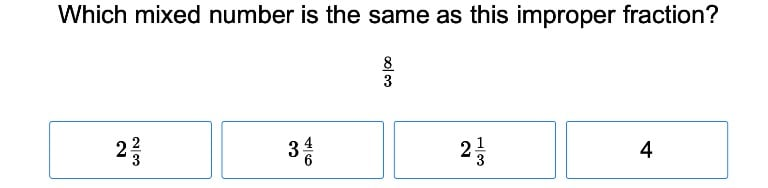After this final exercise in the series, students will be completely comfortable identifying and working with mixed numbers and improper fractions.

## Gem Mining: Converting Improper and Proper Fractions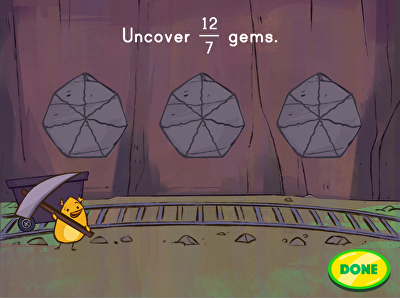Gem mining is a game that teaches converting improper to proper fractions. Students are tasked with helping Birdee uncover the gems in the mine by tapping on the rocks to match the mixed number shown. Students will practice the following fractions: halves, thirds, quarters, fifths, sixths, and eighths. Learning mixed numbers and improper fractions is a key math skill typically covered in the fifth grade.

## Decimal Fractions 2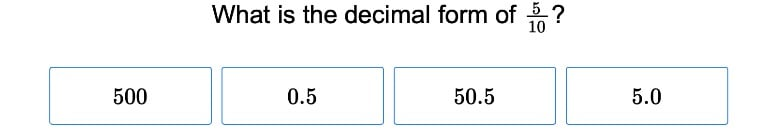Converting decimals to fractions is a lot easier for students once they have worked through this exercise.

## Decimal Fractions 3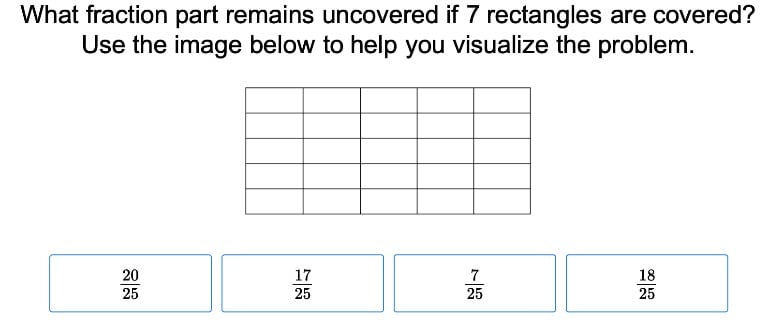Show students how to switch seamlessly back and forth from decimals to fractions to percents with this exercise.

## Area and Unit Fractions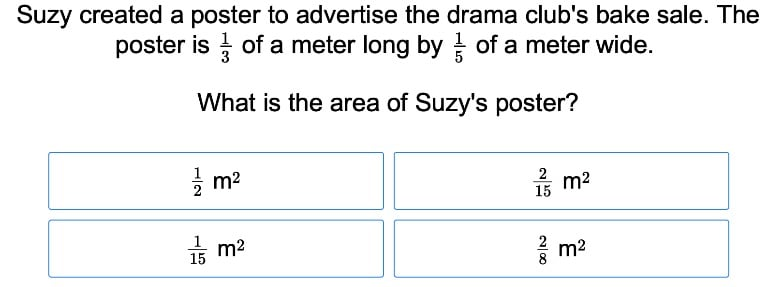Students will understand how to calculate the area of an object with unit fractions as its dimensions after completing this exercise.

## Rectangular Fraction Models and Equivalence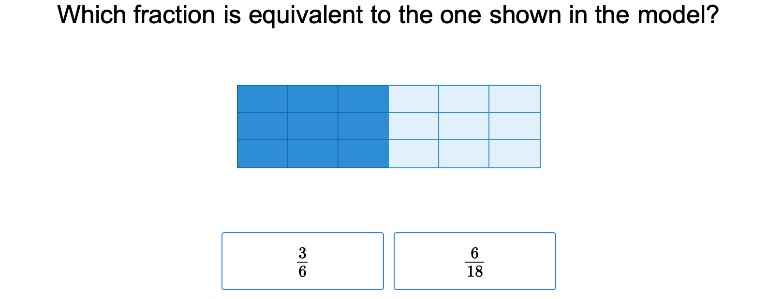If your students need a different way to visualize fractions, show them this exercise on rectangular fraction models and equivalence.